# 11  Univariate volatility

Univariate volatility modelling is designed to provide an in-sample description of the stochastic processes governing volatility. More important, for our purpose here, it also gives one day ahead volatility forecasts which we use for risk calculations.

The mathematics of univariate volatility models are discussed in Chapter 2 of the financial risk forecasting book

We use maximum likelihood methods for estimating volatility models, and use the R package `rugarch` for the actual implementation. See the package documentation for more details, manual and a more detailed vignette. It is developed by Alexios Galanos and is constantly maintained and updated. The development code can be found on github.

This chapter uses a long data sample and all the estimation works without problems. In Chapter 12, we will see what can go wrong with volatility models and how that can be fixed.

## 11.1 Libraries

We used two libraries, `rugarch` for volatility forecasting and `car` for QQ plots.

``````source('common/functions.r')
suppressPackageStartupMessages(library(rugarch))
suppressPackageStartupMessages(library(car))``````

## 11.2 Data

The data we use is returns on the S&P500 index, and for convenience, put them into variable `y`.

``````load('data/data.RData')
y=data\$sp500\$y
y=y-mean(y)``````

## 11.3 What to do with the mean?

One should either remove the mean — de-mean — of the returns, or estimate it along with the other parameters. We can even forecast the mean along with volatility, as we show below.

But since the mean is so small, can usually get away with ignoring it if the sample size is large. Chapter 12 shows how that can fail.

## 11.4 EWMA

Start with the exponentially weighted moving average model, EWMA. We estimate the in-sample EWMA volatility and plot the returns along with +/- two standard deviations.

``````EWMA = vector(length=length(y))
lambda = 0.94
EWMA = var(y)
for (i in 2:length(y)){
EWMA[i] = lambda * EWMA[i-1]+
(1-lambda) * y[i-1] ^2
}
EWMA=sqrt(EWMA)

par(mar=c(2,3.5,1,0))
matplot(
cbind(y,2*EWMA,-2*EWMA),
type='l',
lty=1,
col=c("black","red","red"),
bty='l',
ylab="",
main="SP500 returns with += 2 EWMA sd",
las=1
)``````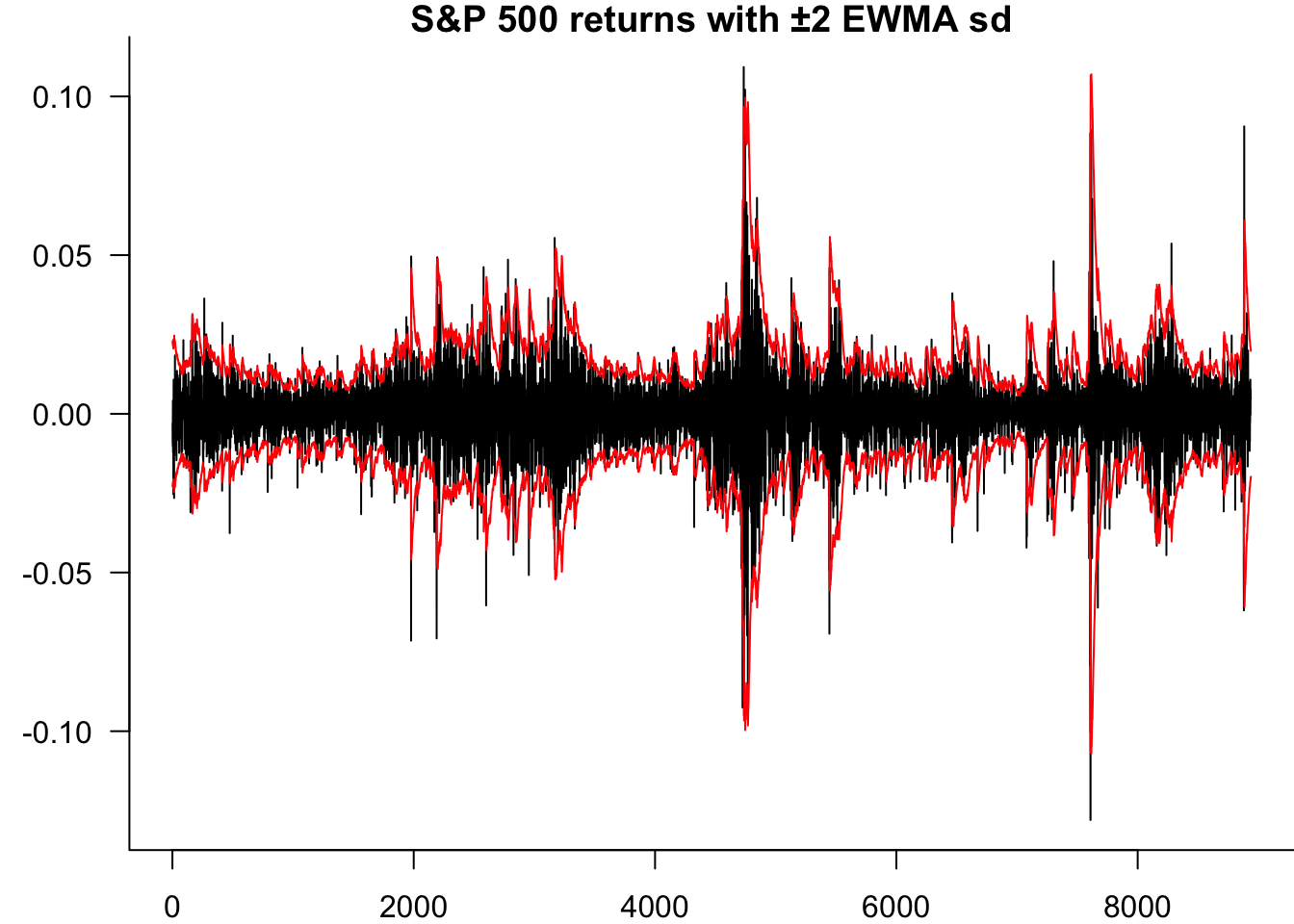## 11.5`rugarch`

### 11.5.1 Setup

To estimate a univariate GARCH model with `rugarch` we need to follow two steps:

1. Create an object of the `uGARCHspec` class which is the specification of the model you want to estimate. This includes the type of model (standard, asymmetric, power, etc.), the GARCH order, the distribution, and model for the mean;
2. Fit the specified model to the data.

### 11.5.2`ugarchspec()`

First let’s take a look at `ugarchspec()`, which is the function to create an instance of the `uGARCHspec` class. The complete syntax with its default values is:

``````ugarchspec(
variance.model = list(
model = "sGARCH",
garchOrder = c(1, 1),
submodel = NULL,
external.regressors = NULL,
variance.targeting = FALSE
),
mean.model = list(
armaOrder = c(1, 1),
include.mean = TRUE,
archm = FALSE,
archpow = 1,
arfima = FALSE,
external.regressors = NULL,
archex = FALSE
),
distribution.model = "norm",
start.pars = list(),
fixed.pars = list()
)``````
``````
*---------------------------------*
*       GARCH Model Spec          *
*---------------------------------*

Conditional Variance Dynamics
------------------------------------
GARCH Model     : sGARCH(1,1)
Variance Targeting  : FALSE

Conditional Mean Dynamics
------------------------------------
Mean Model      : ARFIMA(1,0,1)
Include Mean        : TRUE
GARCH-in-Mean       : FALSE

Conditional Distribution
------------------------------------
Distribution    :  norm
Includes Skew   :  FALSE
Includes Shape  :  FALSE
Includes Lambda :  FALSE ``````

You can check the details of this function and what every argument means by running `?ugarchspec`.

For the purposes of our course, we are going to focus on three arguments of the `ugarchspec()` function:

#### 11.5.2.1`variance.model`

This argument takes a `list` with the specifications of the GARCH model. Its most important components are:

• `model`: Specify the type of model, currently implemented models are “sGARCH”, “fGARCH”, “eGARCH”, “gjrGARCH”, “apARCH” and “iGARCH” and “csGARCH”
• `garchOrder`: Specify the ARCH(q) and GARCH(p) orders

Other components include options to do `variance.targeting`or `external regressors`.

#### 11.5.2.2`mean.model`

This argument takes a `list` with the specifications of the mean model, if assumed to have one. A traditional assumption is that the model has zero mean, in which case we specify
`armaOrder = c(0,0), include.mean = FALSE`
However, it is also common to assume that the mean follows a certain ARMA process.

#### 11.5.2.3`distribution.model`

Here we can specify the conditional density to use for innovations. The default is a normal distribution, but we can specify some others, perhaps the Student-t distribution `std`, or the asymmetric Student-t distribution `sstd`.

### 11.5.3`ugarchfit()`

Once the specification has been created, you can fit this to the data using
`ugarchfit( spec = ..., data = ...)`
The result will be an object of the class `uGARCHfit`, which is a list that contains useful information which will be shown below.

### 11.5.4 Optimiser failure — Hybrid

`ugarchfit()` performs maximum likelihood to fit the specified model to our data. This optimisation problem required a `solver`, which is the numerical method used to maximise the likelihood function. See discussion in Section 12.6. In some cases, the default solver in `ugarchfit()` can fail to converge. Then we need the option `solver = "hybrid"` when fitting the model, since this will try different optimisation methods in case the default one does not converge.

## 11.6 The models

We will put the results into a list called `results()` so we can automatically process them after.

``Results=list()``

### 11.6.1 Gaussian ARCH(1) and no mean

The `garchOrder` in `variance.model` will be set as `c(1,0)`. Note that even if we only have one component, we need to put it inside a `list`.

``````spec.1 = ugarchspec(
variance.model = list(
garchOrder = c(1,0)
),
mean.model = list(
armaOrder = c(0,0),
include.mean = FALSE
)
)``````

We can call `spec.1` to see what is inside:

``spec.1``
``````
*---------------------------------*
*       GARCH Model Spec          *
*---------------------------------*

Conditional Variance Dynamics
------------------------------------
GARCH Model     : sGARCH(1,0)
Variance Targeting  : FALSE

Conditional Mean Dynamics
------------------------------------
Mean Model      : ARFIMA(0,0,0)
Include Mean        : FALSE
GARCH-in-Mean       : FALSE

Conditional Distribution
------------------------------------
Distribution    :  norm
Includes Skew   :  FALSE
Includes Shape  :  FALSE
Includes Lambda :  FALSE ``````

Now we can fit the specified model to our data using the `ugarchfit()` function:

``Results\$ARCH1 = ugarchfit(spec = spec.1, data = y)``
``````Warning in .sgarchfit(spec = spec, data = data, out.sample = out.sample, :
ugarchfit-->warning: solver failer to converge.``````

That fails, need the hybdid solver.

``Results\$ARCH1 = ugarchfit(spec = spec.1, data = y,solver = "hybrid")``

We can check the class of this new object:

``class(Results\$ARCH1)``
`````` "uGARCHfit"
attr(,"package")
 "rugarch"``````
##### 11.6.1.0.1 Printing and plotting

To get all the results, simply do `print(Results\$ARCH1)`
and `plot(Results\$ARCH1)`

Objects from the `uGARCHfit` class have two `slots` (an R term for an object of what is known as the S4). You can think of every slot as a list of components. The two slots are:

1. `@model`;
2. `@fit`.

To access a slot you need to use the syntax: `object@slot`

##### 11.6.1.0.2`@model`

The `@model` slot includes all the information that was needed to estimate the GARCH model. This includes both the model specification and the data. To see every that is included in this slot, you can use the `names()` function:

``names(Results\$ARCH1@model)``
``````  "modelinc"   "modeldesc"  "modeldata"  "pars"       "start.pars"
 "fixed.pars" "maxOrder"   "pos.matrix" "fmodel"     "pidx"
 "n.start"   ``````

And you can access each element with the `\$` sign. For example, let’s see what is inside `pars`: (only rows 7-10).

``Results\$ARCH1@model\$pars[7:10,]``
``````              Level Fixed Include Estimate           LB        UB
omega  8.889786e-05     0       1        1 2.220446e-16 0.1478908
alpha1 4.407729e-01     0       1        1 0.000000e+00 1.0000000
beta   0.000000e+00     0       0        0           NA        NA
gamma  0.000000e+00     0       0        0           NA        NA``````

We have a matrix with all the possible parameters to be used in fitting a GARCH model. We see there is a value of 1 for the parameters that were included in our GARCH specification (`omega`, `alpha1` and `beta1`). The matrix also includes what are the lower and upper bounds for these parameters, which has nothing to do with our particular data, only with what is expected from the model.

We can also see the model description:

``Results\$ARCH1@model\$modeldesc``
``````\$distribution
 "norm"

\$distno
 1

\$vmodel
 "sGARCH"``````

And inside `Results\$ARCH1@model@modeldata` we will find the data vector we have used when fitting the model. You can think of the `@model` slot as everything that R needs to know in order to be able to estimate the model.

##### 11.6.1.0.3`@fit`

Inside the `fit` slot we will find the estimated model, including the coefficients, likelihood, fitted conditional variance, and more. Let’s check everything that is included:

``names(Results\$ARCH1@fit)``
``````  "hessian"         "cvar"            "var"             "sigma"
 "condH"           "z"               "LLH"             "log.likelihoods"
 "residuals"       "coef"            "robust.cvar"     "A"
 "B"               "scores"          "se.coef"         "tval"
 "matcoef"         "robust.se.coef"  "robust.tval"     "robust.matcoef"
 "fitted.values"   "convergence"     "message"         "kappa"
 "persistence"     "timer"           "ipars"           "solver"         ``````

Let’s see the coefficients, along with their standard errors, in `matcoef`. There are 2 ways to do that:

``Results\$ARCH1@fit\$matcoef``
``````           Estimate   Std. Error  t value Pr(>|t|)
omega  8.889786e-05 2.506444e-06 35.46773        0
alpha1 4.407729e-01 3.359505e-02 13.12017        0``````
``coef(Results\$ARCH1)``
``````       omega       alpha1
8.889786e-05 4.407729e-01 ``````

We can also see the log-likelihood. Again two ways

``Results\$ARCH1@fit\$LLH``
`` 15519.61``
``likelihood(Results\$ARCH1)``
`` 15519.61``

This makes it easy to extract the estimated conditional volatility:

``plot(Results\$ARCH1@fit\$sigma, type = "l")``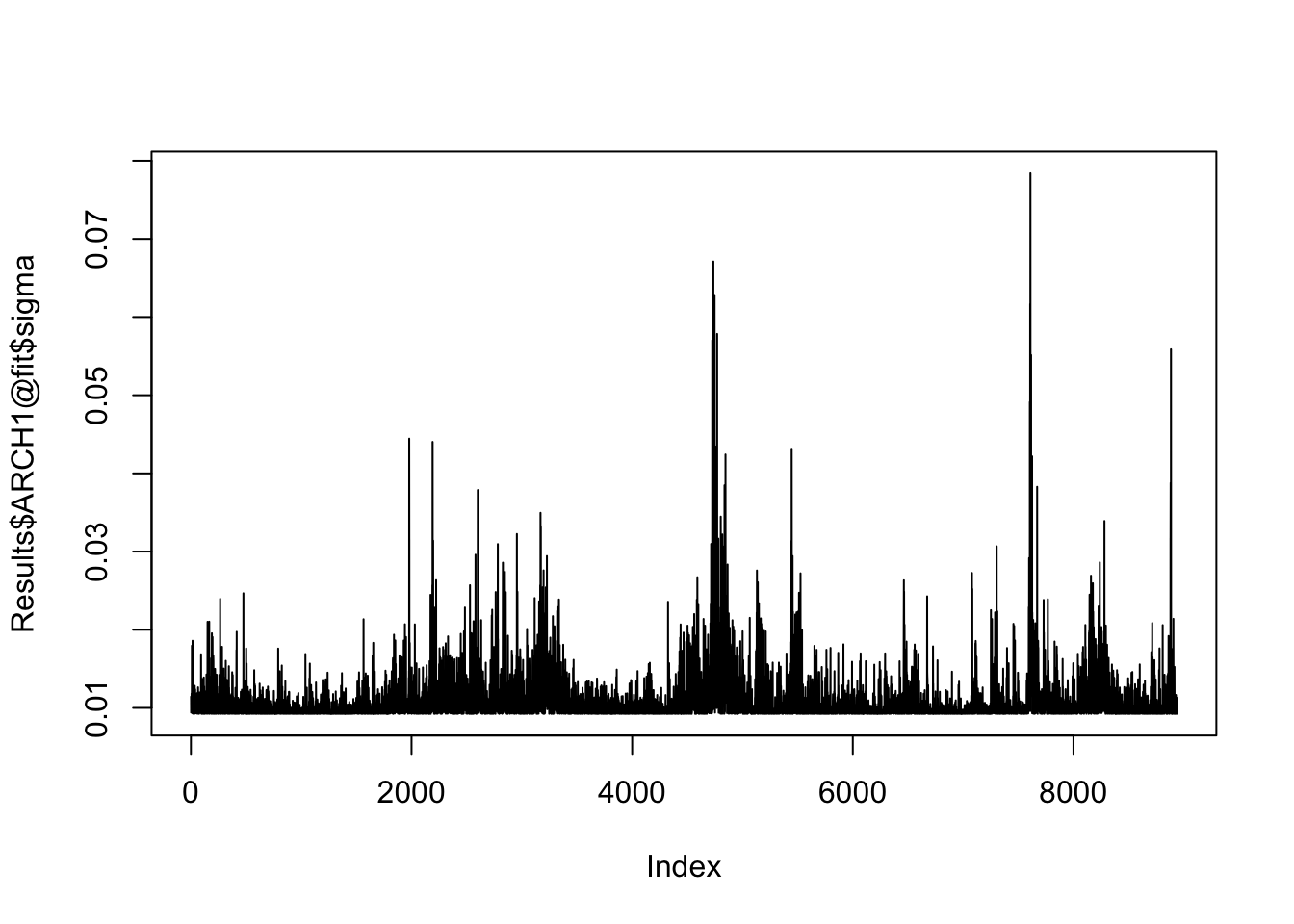### 11.6.2 Gaussian GARCH(1,1)

``````spec.2 = ugarchspec(
variance.model = list(garchOrder = c(1,1)),
mean.model = list(armaOrder = c(0,0), include.mean = FALSE)
)
Results\$GARCH11 = ugarchfit(spec = spec.2, data = y)
Results\$GARCH11@fit\$matcoef``````
``````           Estimate   Std. Error   t value    Pr(>|t|)
omega  2.459018e-06 7.645007e-07  3.216502 0.001297638
alpha1 1.238915e-01 9.913236e-03 12.497585 0.000000000
beta1  8.557904e-01 1.073671e-02 79.706952 0.000000000``````

### 11.6.3 tGARCH(1,1)

We can see that the coefficients now include `shape`, which is the estimated degrees of freedom:

``````spec.3 = ugarchspec(
variance.model = list(garchOrder = c(1,1)),
mean.model = list(armaOrder = c(0,0), include.mean = FALSE),
distribution.model = "std"
)
Results\$tGARCH11 = ugarchfit(spec = spec.3, data = y)
Results\$tGARCH11@fit\$matcoef``````
``````           Estimate   Std. Error    t value     Pr(>|t|)
omega  1.505701e-06 1.959878e-06  0.7682627 4.423311e-01
alpha1 1.240716e-01 3.451712e-02  3.5944939 3.250227e-04
beta1  8.706782e-01 3.112403e-02 27.9744687 0.000000e+00
shape  6.061799e+00 8.255397e-01  7.3428314 2.091660e-13``````

### 11.6.4 Asymmetric tGARCH(1,1)

We can have a skew Student-t conditional distribution.

``````spec.4 = ugarchspec(
variance.model = list(garchOrder = c(1,1)),
mean.model = list(armaOrder = c(0,0), include.mean = FALSE),
distribution.model = "sstd"
)
Results\$atGARCH11 = ugarchfit(spec = spec.4, data = y)
Results\$atGARCH11@fit\$matcoef``````
``````           Estimate   Std. Error    t value     Pr(>|t|)
omega  1.494685e-06 1.844169e-06  0.8104923 4.176573e-01
alpha1 1.227309e-01 3.209377e-02  3.8241355 1.312318e-04
beta1  8.710876e-01 2.926484e-02 29.7656720 0.000000e+00
skew   8.770661e-01 1.669387e-02 52.5381991 0.000000e+00
shape  6.481471e+00 9.600333e-01  6.7512983 1.465272e-11``````

### 11.6.5 Gaussian apARCH model

The parameter list will now include `gamma1` and `delta`, which are part of the apARCH model specification:

``````spec.5 = ugarchspec(
variance.model = list(model = "apARCH"),
mean.model = list(armaOrder = c(0,0), include.mean = FALSE),
)

Results\$APGARCH11 = ugarchfit(spec = spec.5, data = y)
Results\$APGARCH11@fit\$matcoef``````
``````           Estimate   Std. Error    t value   Pr(>|t|)
omega  1.453484e-07 4.735252e-07  0.3069496 0.75888173
alpha1 6.084138e-02 3.590656e-02  1.6944363 0.09018241
beta1  8.728381e-01 4.150876e-02 21.0278064 0.00000000
gamma1 4.460231e-01 1.840133e-01  2.4238627 0.01535641
delta  2.554894e+00 5.051892e-02 50.5730029 0.00000000``````

### 11.6.6 t-apARCH model

``````spec.6 = ugarchspec(
variance.model = list(model = "apARCH"),
mean.model = list(armaOrder = c(0,0), include.mean = FALSE),
distribution.model = "std"
)
Results\$tAPGARCH11 = ugarchfit(spec = spec.6, data = y)
Results\$tAPGARCH11@fit\$matcoef``````
``````           Estimate   Std. Error     t value   Pr(>|t|)
omega  0.0002525432 0.0001215195    2.078211 0.03768989
alpha1 0.1027524046 0.0092548707   11.102522 0.00000000
beta1  0.8969715700 0.0097044547   92.428848 0.00000000
gamma1 0.9999999900 0.0003535216 2828.681436 0.00000000
delta  1.0065891350 0.0907703454   11.089405 0.00000000
shape  6.5133573866 0.5743629275   11.340142 0.00000000``````

### 11.6.7 Asymmetric t-apARCH model

``````spec.7 = ugarchspec(
variance.model = list(model = "apARCH"),
mean.model = list(armaOrder = c(0,0), include.mean = FALSE),
distribution.model = "sstd"
)
Results\$atAPGARCH11 = ugarchfit(spec = spec.7, data = y)
Results\$atAPGARCH11@fit\$matcoef``````
``````           Estimate   Std. Error    t value   Pr(>|t|)
omega  0.0002902321 0.0001322079    2.19527 0.02814421
alpha1 0.1035077895 0.0095115201   10.88236 0.00000000
beta1  0.8975209454 0.0101043456   88.82524 0.00000000
gamma1 0.9999999900 0.0003833587 2608.52302 0.00000000
delta  0.9780618429 0.0842650532   11.60697 0.00000000
skew   0.8487325626 0.0161120763   52.67680 0.00000000
shape  7.0382213920 0.6802275855   10.34686 0.00000000``````

### 11.6.8 Asymmetric t-apARCH model with ARMA(1,1) mean

``````spec.8 = ugarchspec(
variance.model = list(model = "apARCH"),
mean.model = list(armaOrder = c(1,1), include.mean = TRUE),
distribution.model = "sstd"
)
Results\$Mean.atAPGARCH11 = ugarchfit(spec = spec.8, data = y,solver = "hybrid")
Results\$Mean.atAPGARCH11@fit\$matcoef``````
``````            Estimate   Std. Error       t value     Pr(>|t|)
mu     -8.298863e-17 9.366160e-05 -8.860476e-13 1.000000e+00
ar1     4.146306e-01 5.708996e-02  7.262759e+00 3.792522e-13
ma1    -4.871041e-01 5.568657e-02 -8.747245e+00 0.000000e+00
omega   3.511539e-08 2.485181e-06  1.412991e-02 9.887263e-01
alpha1  1.034993e-01 1.069228e-02  9.679819e+00 0.000000e+00
beta1   9.220933e-01 7.358088e-03  1.253170e+02 0.000000e+00
gamma1  5.290305e-01 7.168919e-02  7.379502e+00 1.589839e-13
delta   1.294503e+00 1.373357e-01  9.425829e+00 0.000000e+00
skew    8.448373e-01 1.631212e-02  5.179198e+01 0.000000e+00
shape   5.772091e+00 4.773574e-01  1.209176e+01 0.000000e+00``````

## 11.7 Summary

We can put all the results into a dataframe

``````r=Results[[length(Results)]]

df=data.frame(c(likelihood(r),r@fit\$timer,coef(r)))
rownames(df)[1:2]=c("log.likelihood","time")
rownames(df)[3:dim(df)]=names(coef(r))
names(df)=names(Results)[length(Results)]

for(i in 1:length(Results)){
r=Results[[i]]
x=c(likelihood(r),r@fit\$timer,coef(r))
x=data.frame(c(likelihood(r),r@fit\$timer,coef(r)))
rownames(x)[1:2]=c("log.likelihood","time")
rownames(x)[3:dim(x)]=names(coef(r))

df[[names(Results)[i]]]=NA
df[rownames(x),names(Results)[i]]=x

}
df=round(df,3)
df=df[,names(Results)]

df.summary=df``````

### 11.7.1 Issues

#### 11.7.1.1 Sample size

It is important to consider that the more parameter a model has, the more likely it runs into estimation problems, and the more data we need. For example, as a rule of thumb, for a GARCH(1,1) we need at least 500 observations, while for a Student-t GARCH that minimum number can be around 3,000 observations. In general, the more parameters are estimated, the more data is needed to be confident in the estimation. We see an example of this in Chapter 12.

## 11.8 Diagnostics

### 11.8.1 Likelihood ratio test

The likelihood ratio (LR) test compares two models based on the ratio of their likelihoods,

``````# Perform the LR test
LR_statistic = 2*(likelihood(Results\$GARCH11)-likelihood(Results\$ARCH1))
p_value  = 1 - pchisq(LR_statistic, df = 1)

cat(" Likelihood of ARCH: ", round(likelihood(Results\$ARCH1),1), "\n",
"Likelihood of GARCH:", round(likelihood(Results\$GARCH11),1), "\n",
"2 * (Lu - Lr): ", round(LR_statistic,2), "\n",
"p-value:", p_value)``````
`````` Likelihood of ARCH:  15519.6
Likelihood of GARCH: 16455.3
2 * (Lu - Lr):  1871.3
p-value: 0``````

We find a p-value of 0, meaning that we have enough evidence to reject the null hypothesis that the two models are the same. The GARCH model has a significantly larger likelihood than the ARCH model.

### 11.8.2 Residual analysis

#### 11.8.2.1 GARCH

``````residuals=y/Results\$GARCH11@fit\$sigma
par(mar=c(2,4,2,0))
plot(residuals,type='l',bty='l',las=1)``````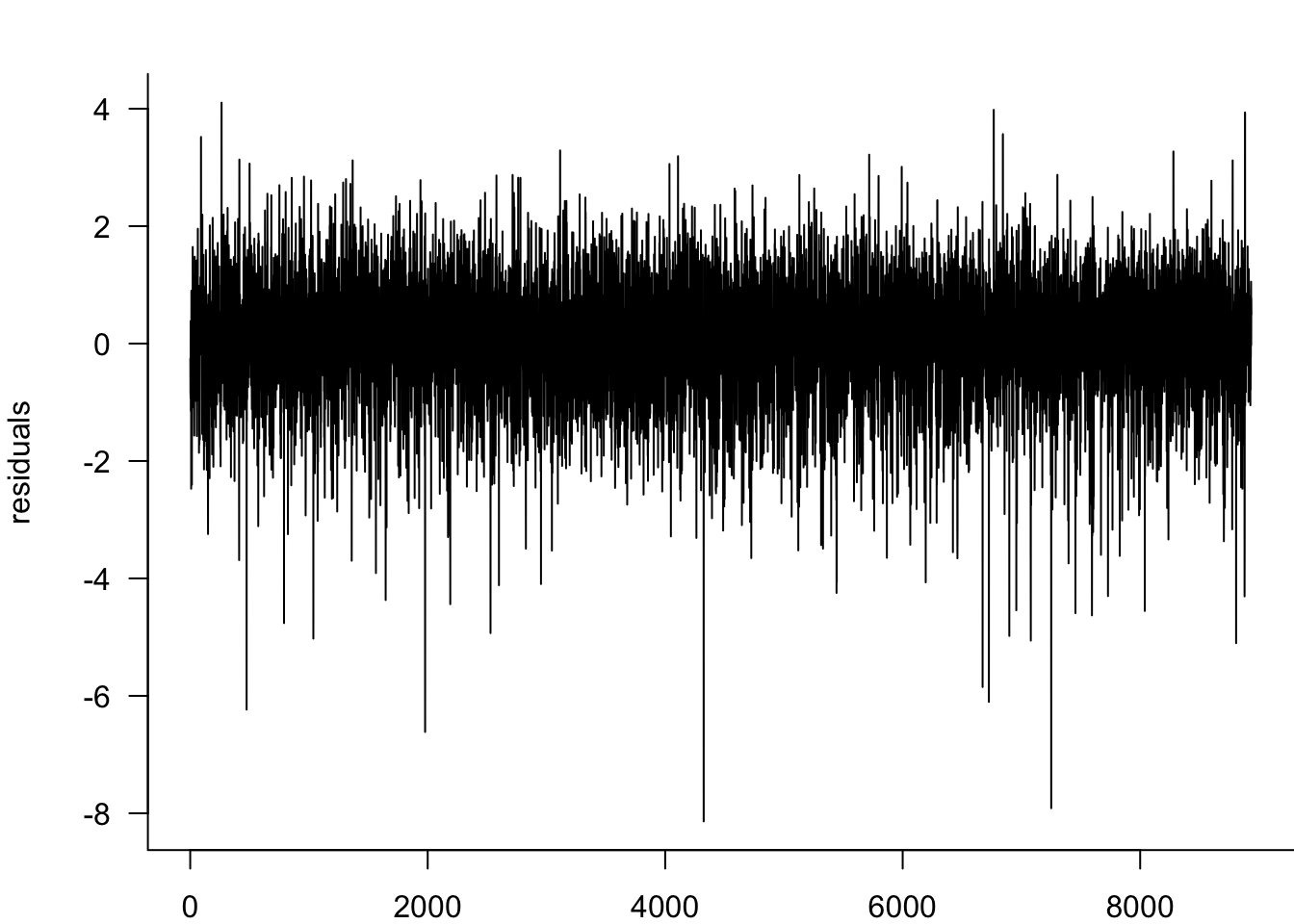``myACF(residuals,main="ACF of SP-500 returns")``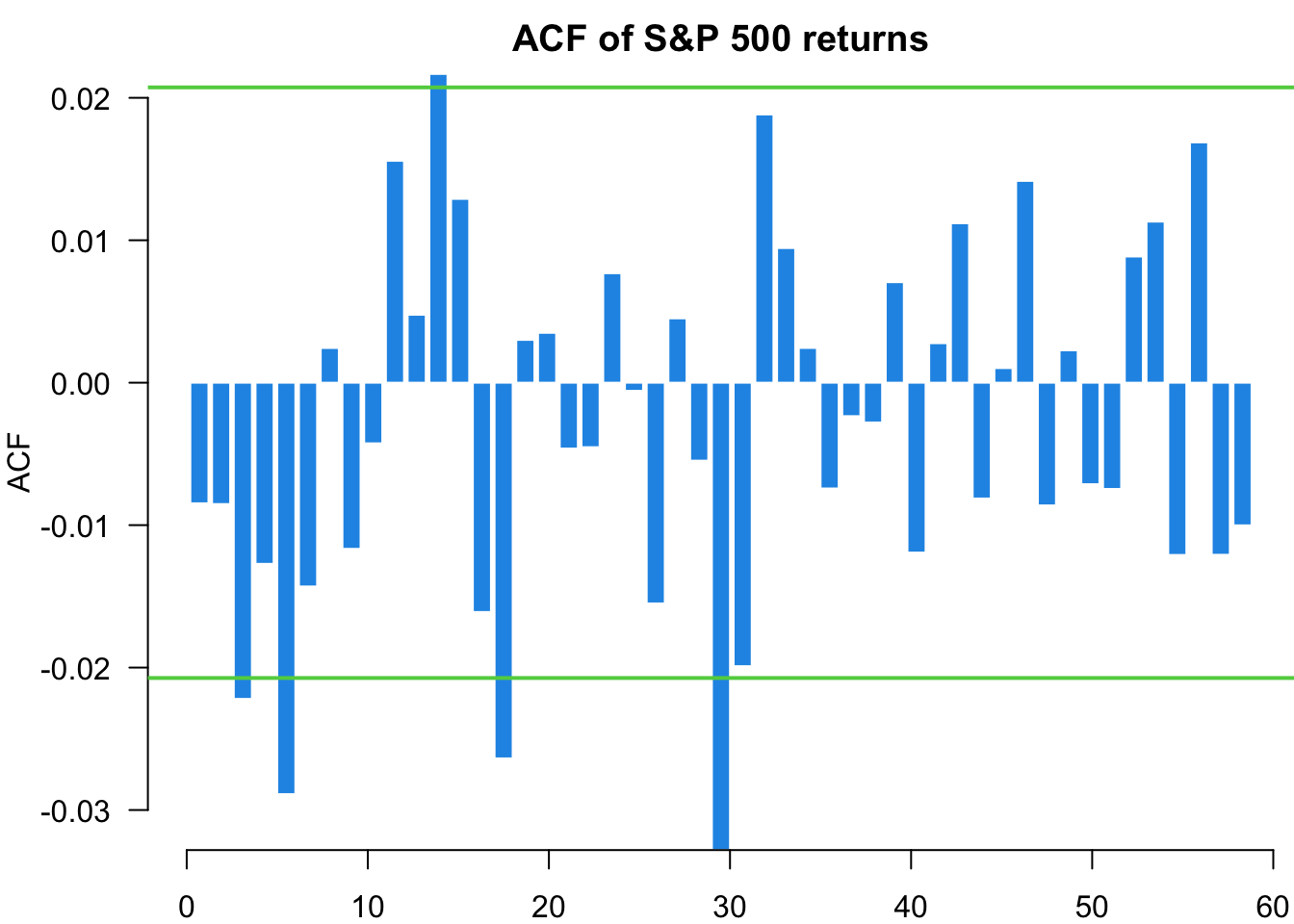``myACF(residuals^2,main="ACF of squared SP-500 returns")``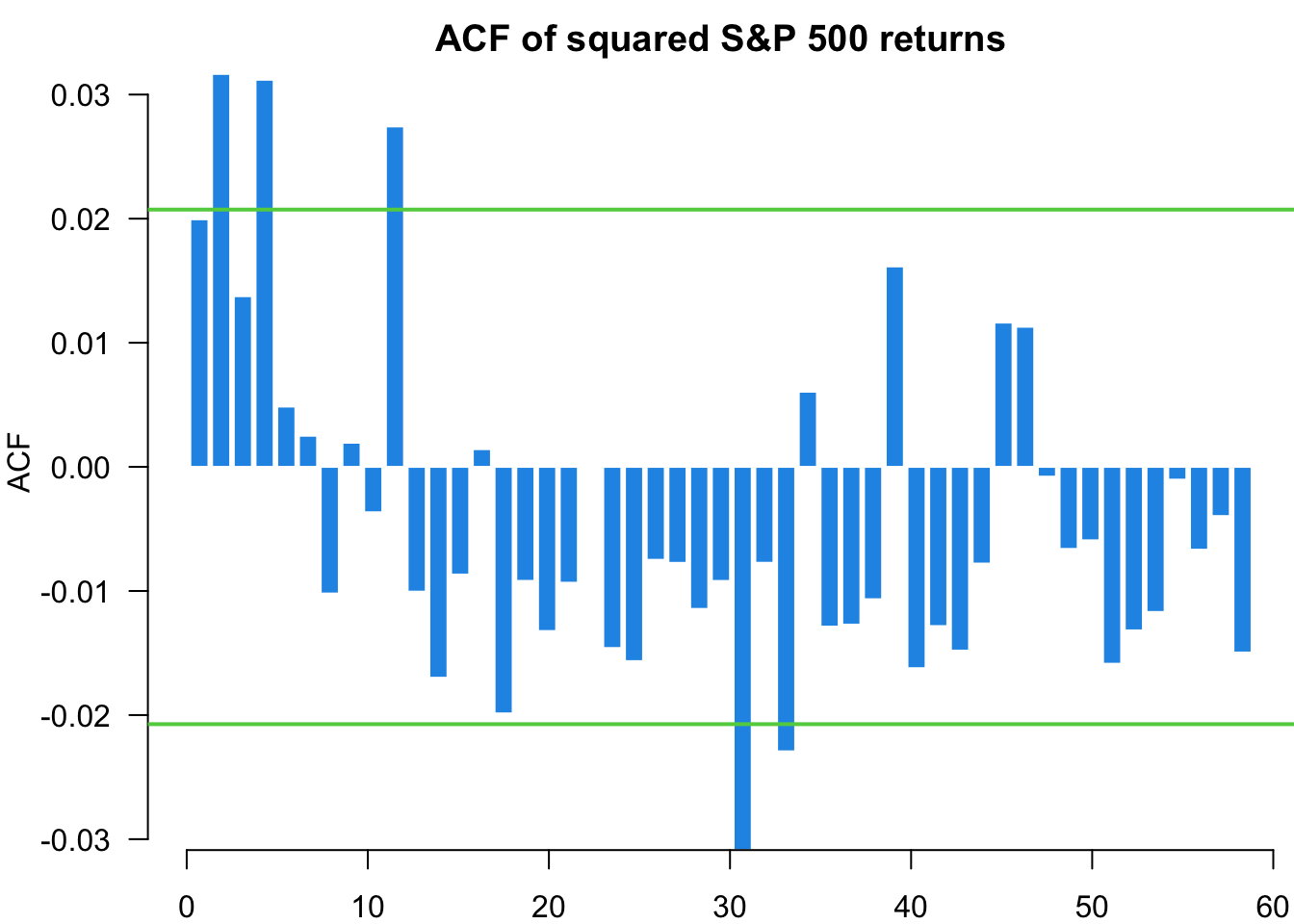``x=qqPlot(residuals, distribution = "norm", envelope = FALSE,main="Normal QQ of SP-500 returns")``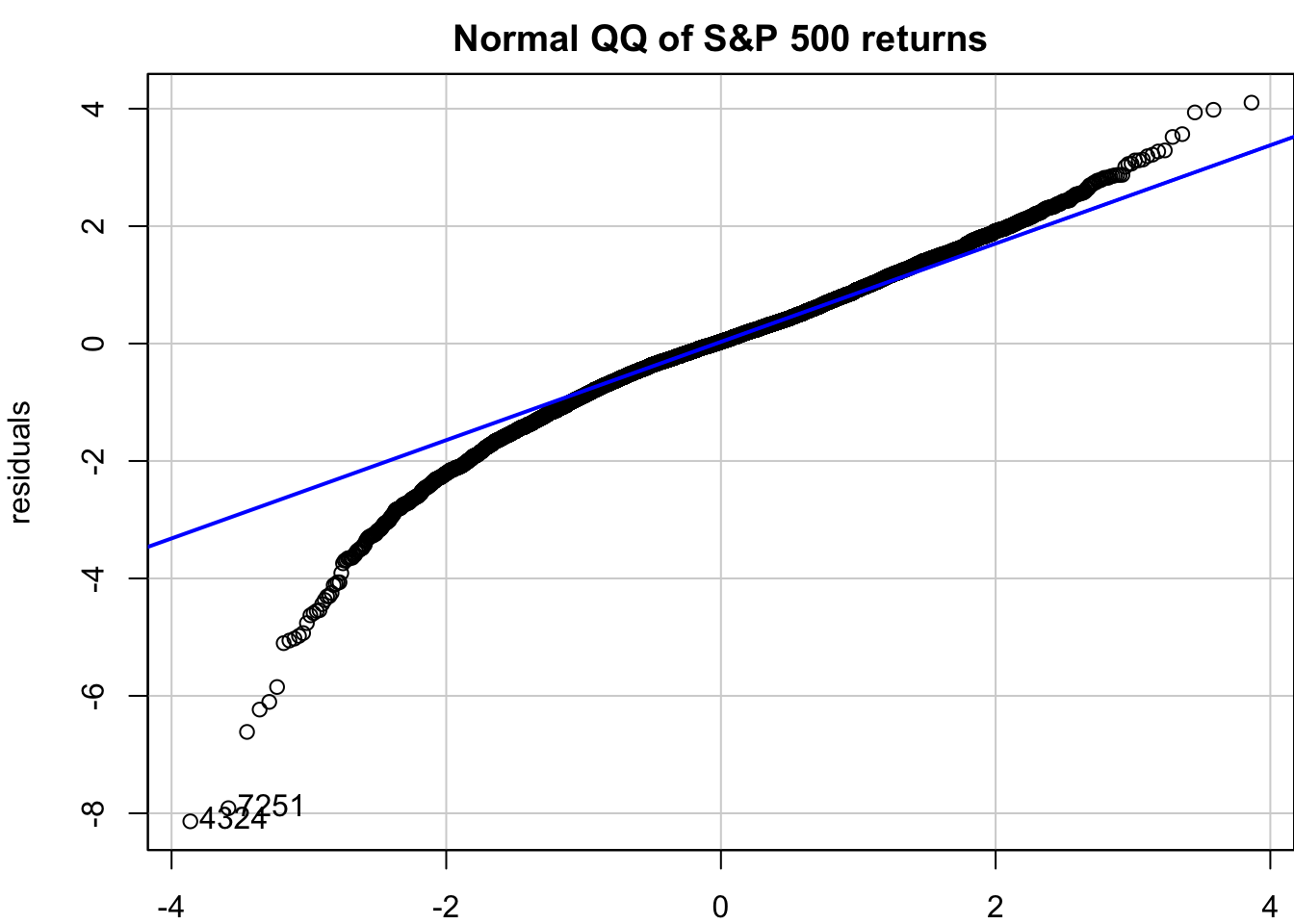## 11.9 Monte Carlo analysis of GARCH models

### 11.9.1 Using `ugarchsim()`

#### 11.9.1.1 Gaussian

``````s=ugarchsim(Results\$GARCH11, n.sim = 5000)
par(mar=c(2,4,2,0))
plot(y,type='l',bty='l',las=1,main="SP500")````````plot(s@simulation\$seriesSim,type='l',bty='l',las=1,main="Simulated GARCH SP500")``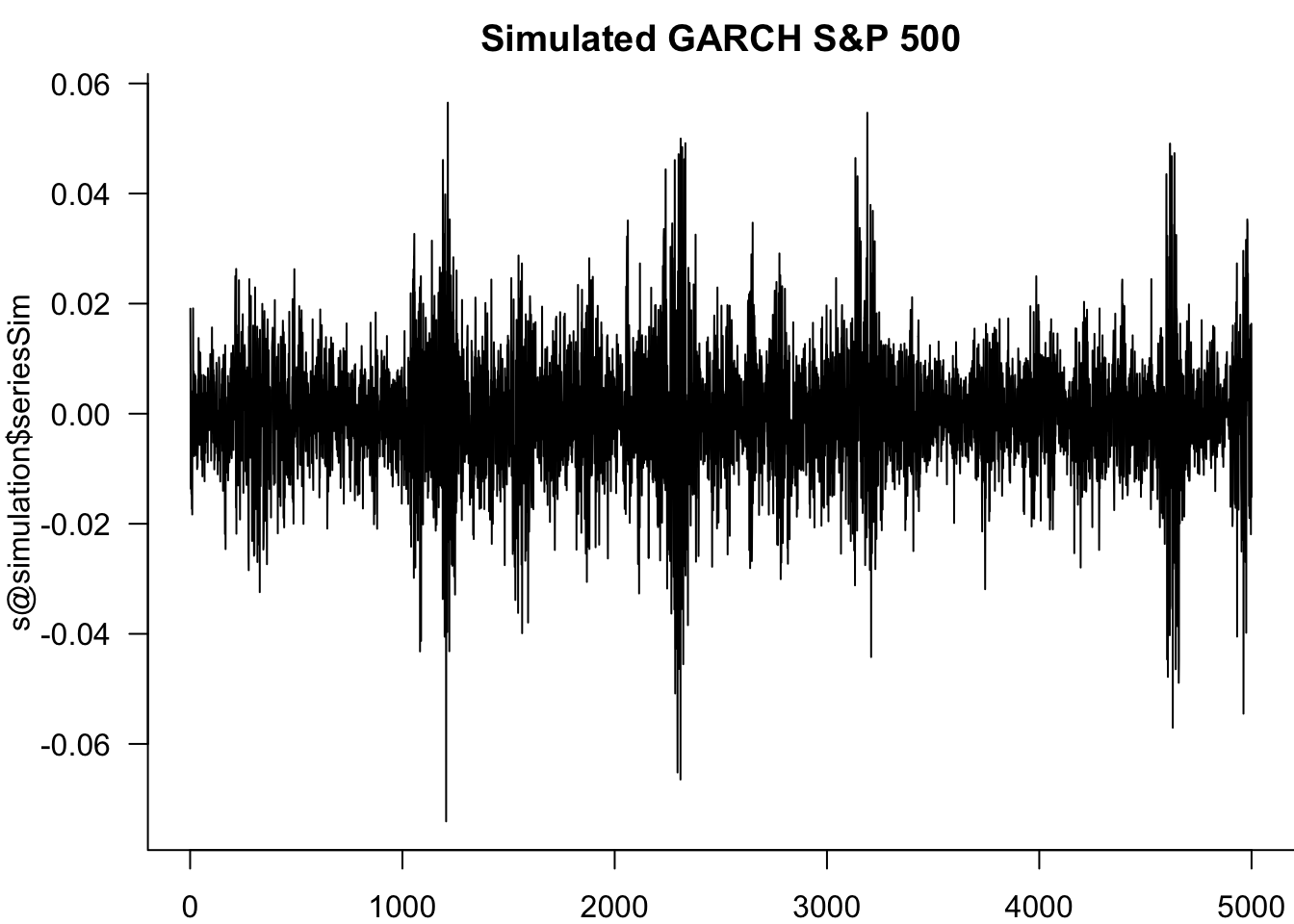``````s=ugarchsim(Results\$tGARCH11, n.sim = 5000)
par(mar=c(2,3,2,0))
plot(y,type='l',bty='l',las=1,main="SP500")``````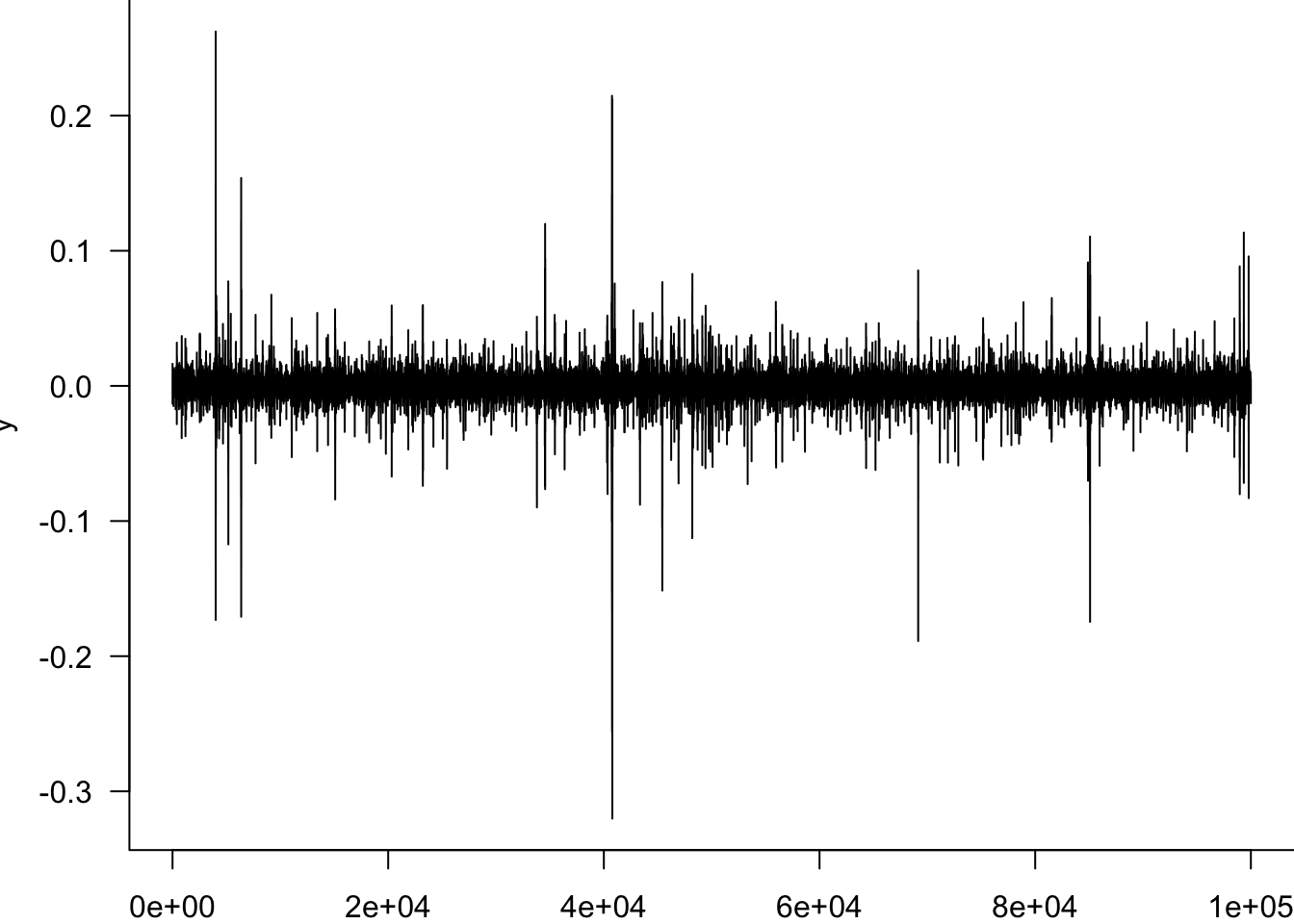``plot(s@simulation\$seriesSim,type='l',bty='l',las=1,main="Simulated tGARCH SP500")``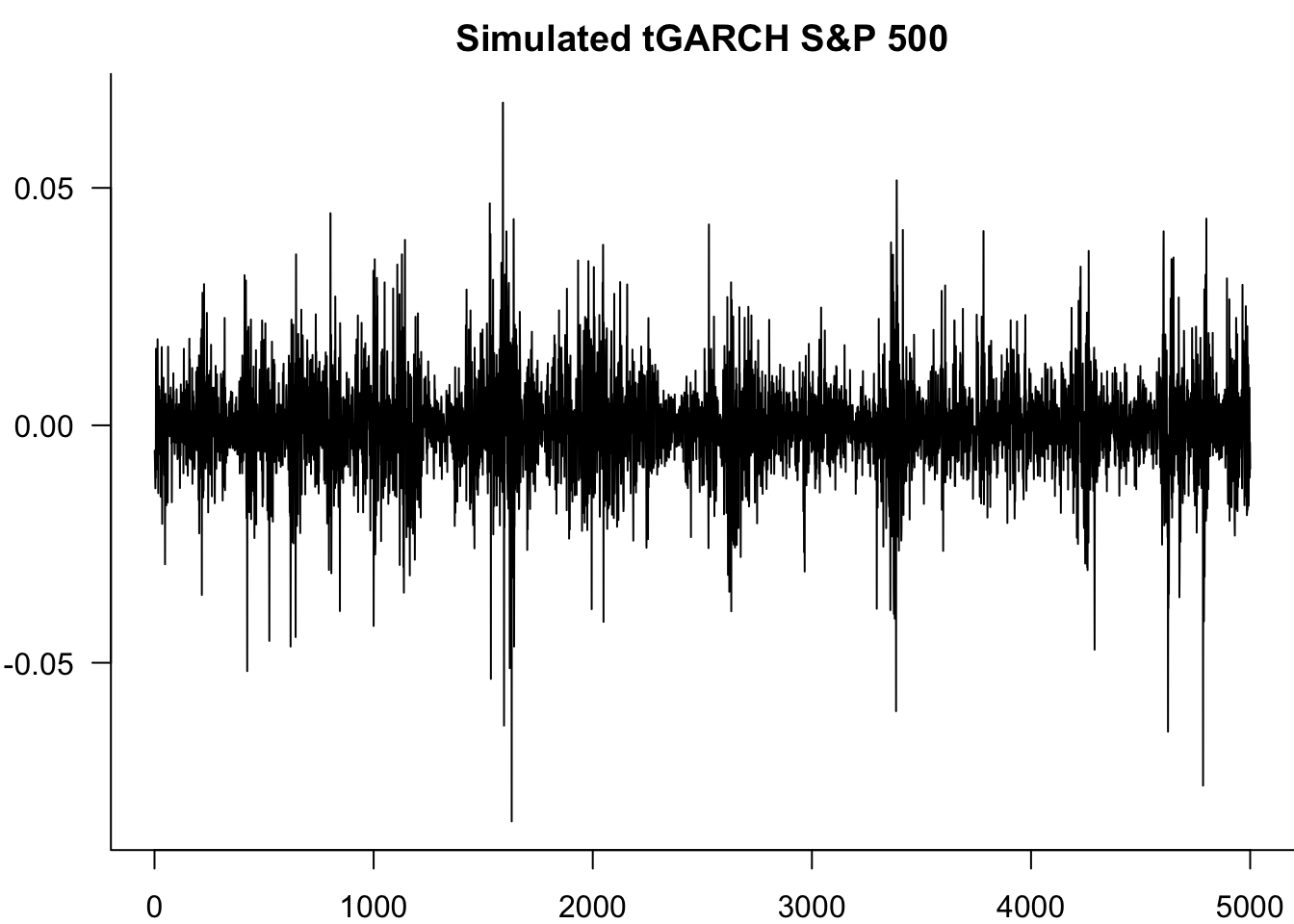#### 11.9.1.2 Simulation and estimation

``````N=5
for(n in 1:N){
s=ugarchsim(Results\$GARCH11, n.sim = 5000)
Results\$GARCH11 = ugarchfit(spec = spec.2, data = s@simulation\$seriesSim)
print(Results\$GARCH11@fit\$matcoef)
}``````
``````           Estimate   Std. Error   t value   Pr(>|t|)
omega  3.378344e-06 1.710221e-06  1.975385 0.04822451
alpha1 1.065208e-01 9.727697e-03 10.950260 0.00000000
beta1  8.562490e-01 1.448400e-02 59.116874 0.00000000
Estimate   Std. Error   t value    Pr(>|t|)
omega  3.374407e-06 1.127587e-06  2.992591 0.002766201
alpha1 1.125439e-01 9.938571e-03 11.323949 0.000000000
beta1  8.565786e-01 1.211022e-02 70.731904 0.000000000
Estimate   Std. Error   t value    Pr(>|t|)
omega  2.897911e-06 9.758330e-07  2.969679 0.002981112
alpha1 1.019786e-01 9.272800e-03 10.997609 0.000000000
beta1  8.680405e-01 1.146641e-02 75.702921 0.000000000
Estimate   Std. Error   t value    Pr(>|t|)
omega  3.117605e-06 1.122498e-06  2.777381 0.005479887
alpha1 9.381668e-02 9.771660e-03  9.600895 0.000000000
beta1  8.773633e-01 1.223240e-02 71.724522 0.000000000
Estimate   Std. Error   t value    Pr(>|t|)
omega  2.976626e-06 1.050824e-06  2.832660 0.004616252
alpha1 1.056688e-01 1.087993e-02  9.712271 0.000000000
beta1  8.699828e-01 1.275530e-02 68.205612 0.000000000``````

### 11.9.2 Manual simulation

#### 11.9.2.1 GARCH(1,1)

``````S=1e3
omega=1e-6
alpha=0.15
beta=0.8

N=10000
set.seed(888)
Res=NULL
for(n in 1:N){
eps=rnorm(S)
y=rep(NA,S)
sigma2 = omega/(1-alpha-beta)

y=eps*sqrt(sigma2)
for(i in 2:S){
sigma2= omega+alpha*y[i-1]^2 + beta * sigma2
y[i]=eps[i]*sqrt(sigma2)
}

}
par(mar=c(2,3.5,0,0))
plot(y,type='l',bty='l',las=1)``````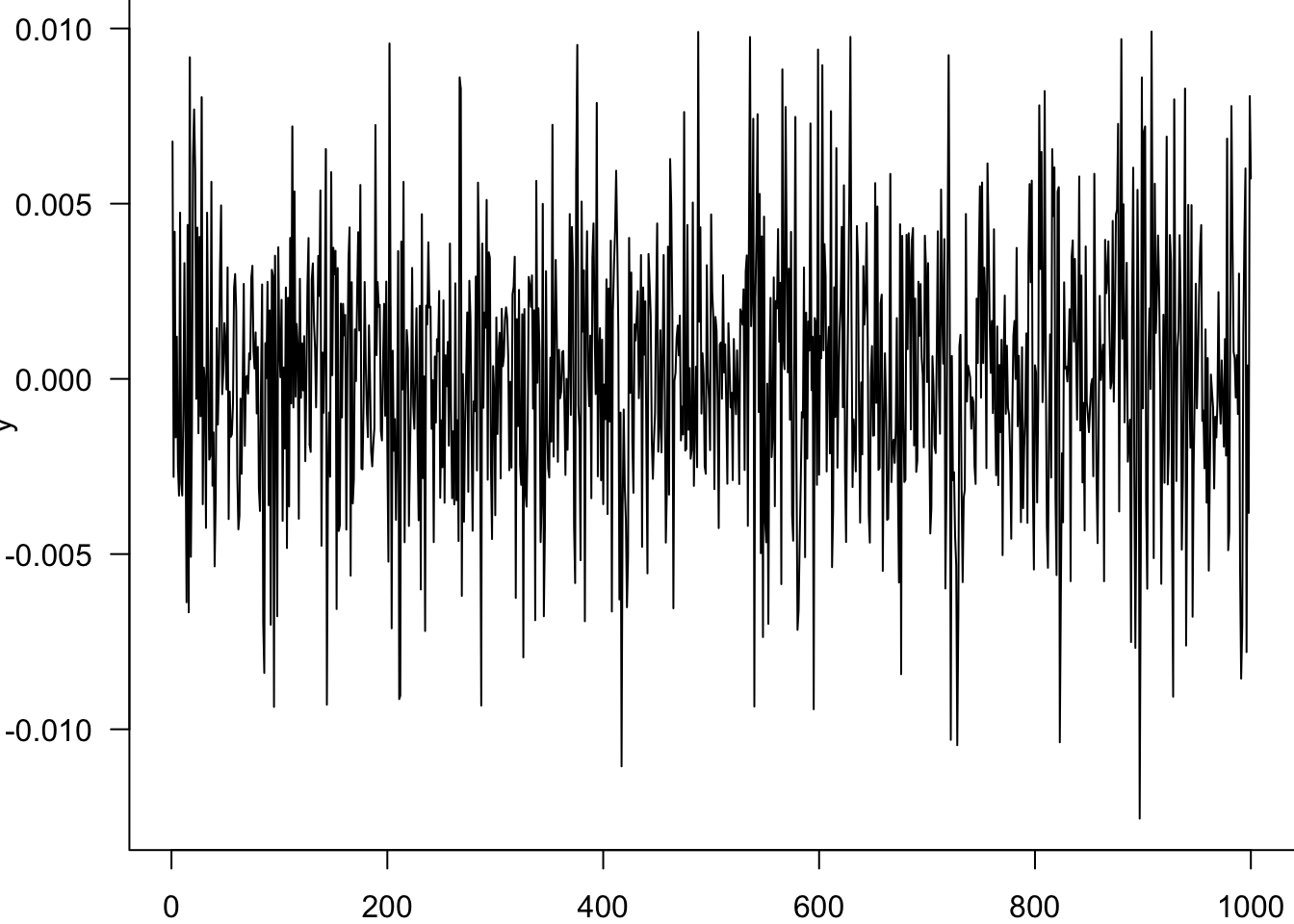#### 11.9.2.2 tGARCH

``````df=6
omega=0.000001
alpha=0.15
beta=0.75
N=1
S=1e5
set.seed(888)
for(n in 1:N){
eps=rt(S,df=df)
y=rep(NA,S)
sigma2 = omega/(1-alpha-beta)

y=eps*sqrt(sigma2)
for(i in 2:S){
sigma2 = omega + alpha*y[i-1]^2 + beta * sigma2
y[i]=eps[i]*sqrt(sigma2)
}
}
par(mar=c(2,3.5,0,0))
plot(y,type='l',bty='l',las=1)  ``````## 11.10 Exercise

We estimated univariate models using eight different specifications in this chapter. Conduct pairwise likelihood ratio tests for all eight model specifications. Comment on the results.

``````# Answer
# df.summary refers to the data frame generated in the summary section
library(combinat)
cb=combn(1:8, 2)

rst=c()
for (i in 1:ncol(cb)){
idx=cb[,i]
lr.tmp=2*abs(df.summary[1,idx]-df.summary[1,idx])
pval.tmp=1-pchisq(lr.tmp, df=1)
rst.tmp=c(lr.tmp, pval.tmp)
rst=rbind(rst, rst.tmp)
}
colnames(rst)=c("LR Stat", "p-value")
rownames(rst)=1:nrow(rst)
rst
which(rst[,2]>0.05)

# We reject the null hypothesis that two models are the same for all pairs.``````